Question

# How to dynamically allocate a 2D array if the number of row is not defined? (in...

How to dynamically allocate a 2D array if the number of row is not defined? (in C-Language)

Need an example code.

By not defined , you mean they will be known at run-time ,

Well if thats the case , here we go!!

#include <stdio.h>
#include <stdlib.h>

int main()
{

//define variables
int row,column;

//input values
scanf("%d",&row);
scanf("%d",&column);

//allocate 2D array

int (*arr)[row] = malloc(row * sizeof(*arr));

//check it

for(int i = 0; i < row; i++) {
for(int j = 0; j < column; j++) {
arr[i][j] = 0;
printf("%d",arr[i][j]);
printf(" ");
}
printf("\n");
}

return 0;
}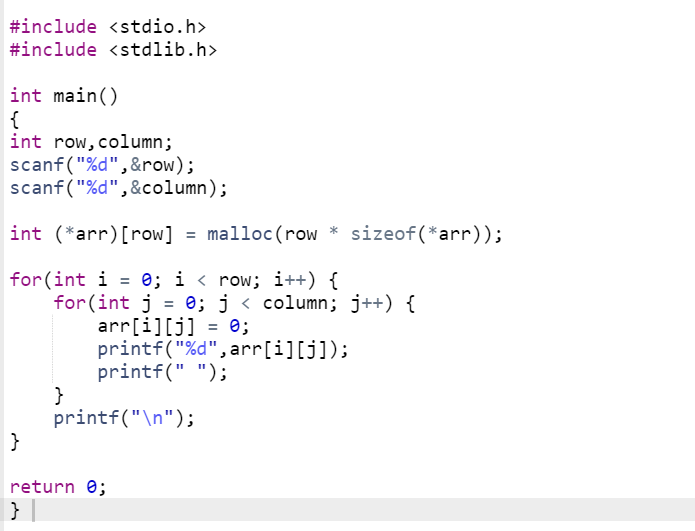Inputs - 2 3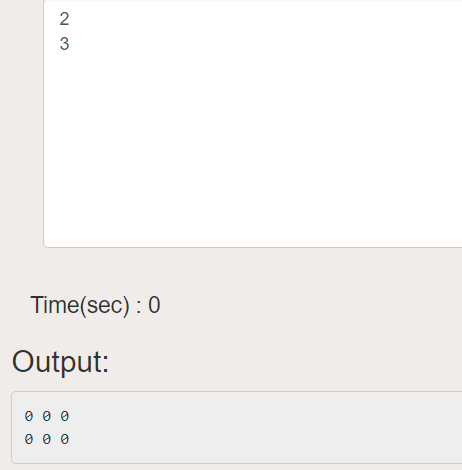#### Earn Coins

Coins can be redeemed for fabulous gifts.

Similar Homework Help Questions
• ### WRITE THE NECESSARY C++ STATEMENTS 1. (10 points) Allocate memory dynamically for an int array with...WRITE THE NECESSARY C++ STATEMENTS 1. (10 points) Allocate memory dynamically for an int array with 100 elements. Next, assign the value of 1 to all the elements of the array and, finally, delete the array.

• ### C Language Write the code that dynamically allocates an array of struct objects based on a...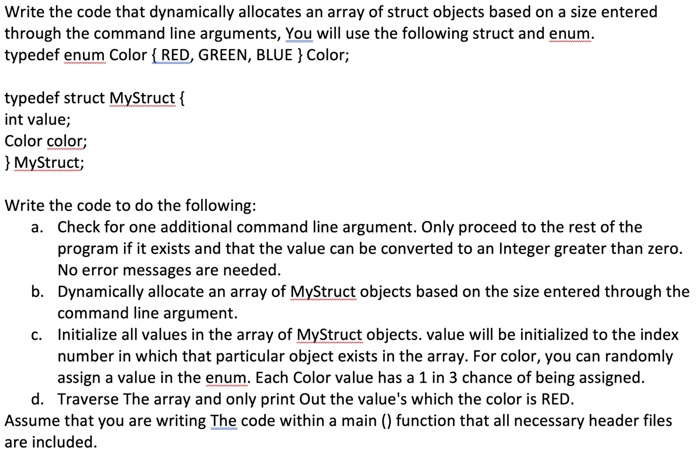C Language Write the code that dynamically allocates an array of struct objects based on a size entered through the command line arguments, You will use the following struct and enum. typedef enum Color { RED, GREEN, BLUE } Color; typedef struct MyStruct { int value; Color color; } MyStruct; Write the code to do the following: a. Check for one additional command line argument. Only proceed to the rest of the program if it exists and that the value...

• ### Write the code to dynamically allocate ONE integer variable using calloc (contiguous allocation) or malloc (memory...

Write the code to dynamically allocate ONE integer variable using calloc (contiguous allocation) or malloc (memory allocation) and have it pointed to by a pointer (of type int * ) named ptr_1. Use ptr_1 to assign the number 7 to that dynamically allocated integer, and in another line use printf to output the contents of that dynamically allocated integer variable. Write the code to dynamically allocate an integer array of length 5 using calloc or malloc and have it pointed...

• ### read it carefully C++ Question: Write a program that dynamically allocates an array large enough to...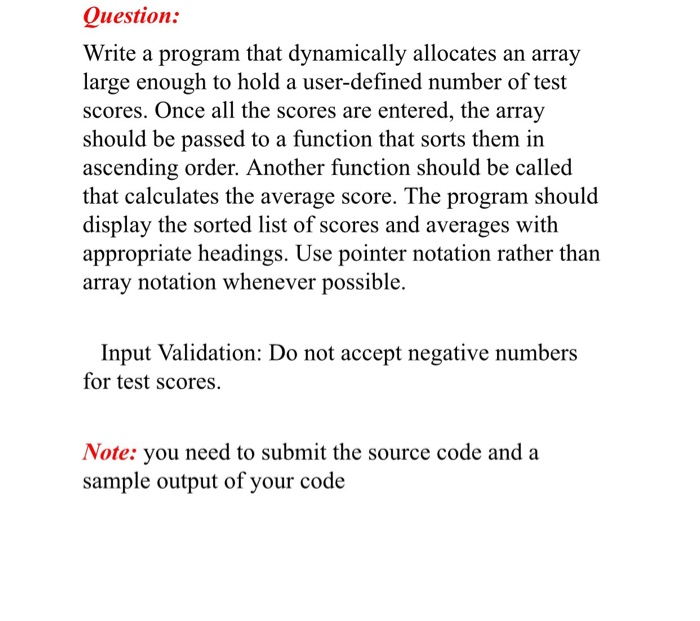read it carefully C++ Question: Write a program that dynamically allocates an array large enough to hold a user-defined number of test scores. Once all the scores are entered, the array should be passed to a function that sorts them in ascending order. Another function should be called that calculates the average score. The program should display the sorted list of scores and averages with appropriate headings. Use pointer notation rather than array notation whenever possible. Input Validation: Do not...

• ### Write a program that dynamically allocates an integer array of size of 20 to hold a...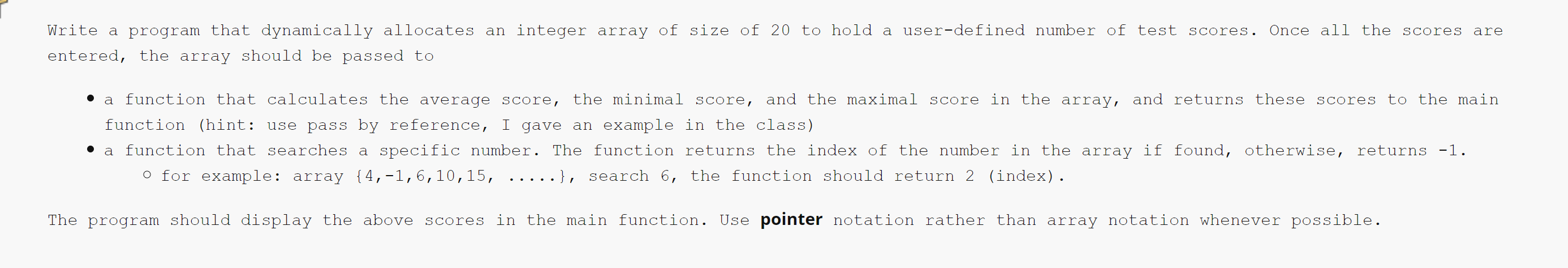Write a program that dynamically allocates an integer array of size of 20 to hold a user-defined number of test scores. Once all the scores are entered, the array should be passed to • a function that calculates the average score, the minimal score, and the maximal score in the array, and returns these scores to the main function (hint: use pass by reference, I gave an example in the class) • a function that searches a specific number. The...

• ### Need help with 2D Array Script

public static double[][] trainNetwork(double[][] trainingSet,double[][] kernelSet, double variance, int nClassifications, double learningRate, int nIterations, Random rng){//Create a new 2D array of weights//number of rows: the number of classifications//number of cols: one larger than the number of rows in the KernelSet//Initialize your new weights array with random doubles in the range//-1.0 inclusive to +1.0 exclusive//Create a new 2D array of inputs for the perceptrons//number of rows: the length of the training set//number of cols: same as the number of cols in...

• ### Create a simple Matrix class with appropriate constructors/destructor. The constructor should dynamically allocate memory for the...

Create a simple Matrix class with appropriate constructors/destructor. The constructor should dynamically allocate memory for the array based on the dimensions provided. this is in C++

• ### Write a function called ZeroCornersSecondRow that sets the corners of a 2D array to zero and...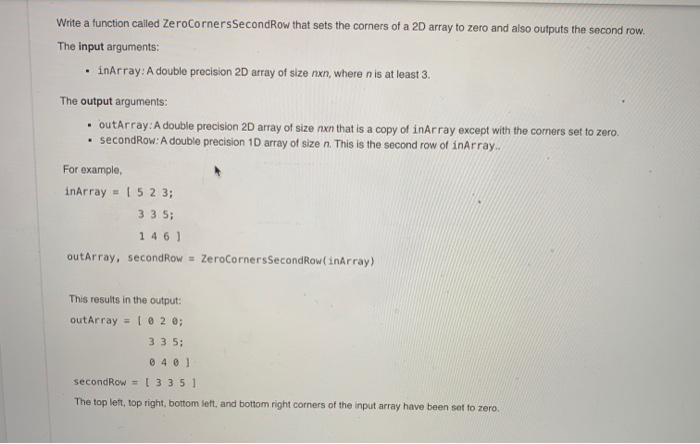Write a function called ZeroCornersSecondRow that sets the corners of a 2D array to zero and also outputs the second row. The input arguments: • inArray: A double precision 2D array of size nxn, where n is at least 3. The output arguments: outArray: A double precision 2D array of size nxn that is a copy of inArray except with the corners set to zero. • secondRow: A double precision 1D array of size n. This is the second row...

• ### 2D array operations

Write a program that creates a two-dimensional array initialized with test data. Use any primitive data type you wish. The program should have the followingmethods.• getTotal - This method should accept a two-dimensional array as its argument and return the total of all the values in the array.• getAverage - This method should accept a two-dimensional array as its argument and return the average of all the values in the array.• getRowTotal. - This method should accept a two-dimensional array...

• ### programming in C a study guide question. how can I access an element of a dynamically...

programming in C a study guide question. how can I access an element of a dynamically allocated array without using [] operator Given ar2 is defined as int** ar2, access ar2[i][j] without using the [] operator. please provide code.